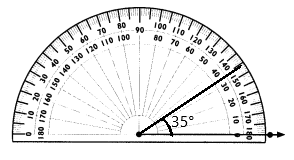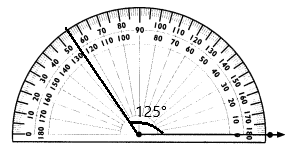# Math in Focus Grade 4 Chapter 9 Practice 2 Answer Key Drawing Angles to 180°

Practice the problems of Math in Focus Grade 4 Workbook Answer Key Chapter 9 Practice 2 Drawing Angles to 180° to score better marks in the exam.

## Math in Focus Grade 4 Chapter 9 Practice 2 Answer Key Drawing Angles to 180°

Use a protractor to draw each angle.

Question 1.
70° using inner scale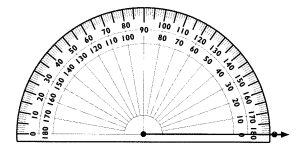We have constructed 70° using inner scale.

Explanation:
Here, we have constructed 70° using inner scale. As the angle opens to the right of the protractor.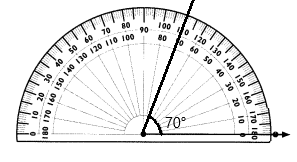Question 2.
147° using outer scale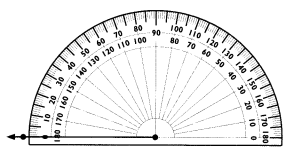We have constructed 147° using outer scale.

Explanation:
Here, we have constructed 147° using outer scale. As the angle opens to the left of the protractor.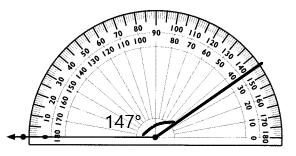Question 3.
35° using outer scale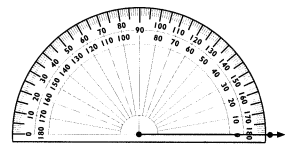We have constructed 35° using outer scale.

Explanation:
Here, we have constructed 35° using outer scale. As the angle opens to the left of the protractor.

Question 4.
108° using outer scale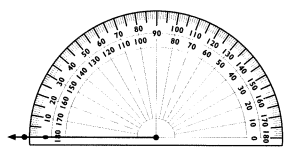We have constructed 108° using outer scale.

Explanation:
Here, we have constructed 108° using outer scale. As the angle opens to the left of the protractor.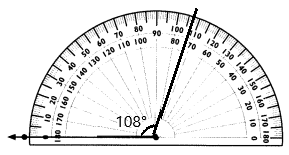Join the marked endpoint of each ray to one of the dots to form an angle with the given value. Then label the angle.

Example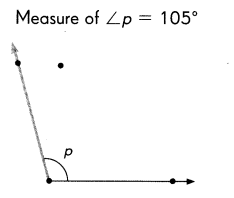Question 5.
Measure of ∠h = 32°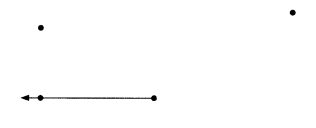Here, we have constructed ∠h = 32°.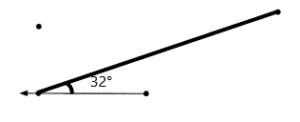Draw an angle that has each measure.

Question 14.
35°